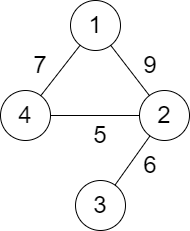##### Welcome to Subscribe On Youtube

Formatted question description: https://leetcode.ca/all/2492.html

# 2492. Minimum Score of a Path Between Two Cities

• Difficulty: Medium.
• Related Topics: .
• Similar Questions: Checking Existence of Edge Length Limited Paths, Checking Existence of Edge Length Limited Paths II.

## Problem

You are given a positive integer n representing n cities numbered from 1 to n. You are also given a 2D array roads where roads[i] = [ai, bi, distancei] indicates that there is a **bidirectional **road between cities ai and bi with a distance equal to distancei. The cities graph is not necessarily connected.

The score of a path between two cities is defined as the **minimum **distance of a road in this path.

Return the **minimum **possible score of a path between cities **1 and **n.

Note:

• A path is a sequence of roads between two cities.

• It is allowed for a path to contain the same road multiple times, and you can visit cities 1 and n multiple times along the path.

• The test cases are generated such that there is at least one path between 1 and n.

Example 1:Input: n = 4, roads = [[1,2,9],[2,3,6],[2,4,5],[1,4,7]]
Output: 5
Explanation: The path from city 1 to 4 with the minimum score is: 1 -> 2 -> 4. The score of this path is min(9,5) = 5.
It can be shown that no other path has less score.


Example 2:Input: n = 4, roads = [[1,2,2],[1,3,4],[3,4,7]]
Output: 2
Explanation: The path from city 1 to 4 with the minimum score is: 1 -> 2 -> 1 -> 3 -> 4. The score of this path is min(2,2,4,7) = 2.


Constraints:

• 2 <= n <= 105

• 1 <= roads.length <= 105

• roads[i].length == 3

• 1 <= ai, bi <= n

• ai != bi

• 1 <= distancei <= 104

• There are no repeated edges.

• There is at least one path between 1 and n.

## Solution (Java, C++, Python)

• class Solution {
private List<int[]>[] g;
private boolean[] vis;
private int ans = 1 << 30;

public int minScore(int n, int[][] roads) {
g = new List[n];
vis = new boolean[n];
Arrays.setAll(g, k -> new ArrayList<>());
for (var e : roads) {
int a = e - 1, b = e - 1, d = e;
}
dfs(0);
return ans;
}

private void dfs(int i) {
for (var nxt : g[i]) {
int j = nxt, d = nxt;
ans = Math.min(ans, d);
if (!vis[j]) {
vis[j] = true;
dfs(j);
}
}
}
}

• class Solution {
public:
int minScore(int n, vector<vector<int>>& roads) {
vector<vector<pair<int, int>>> g(n);
bool vis[n];
memset(vis, 0, sizeof vis);
for (auto& e : roads) {
int a = e - 1, b = e - 1, d = e;
g[a].emplace_back(b, d);
g[b].emplace_back(a, d);
}
int ans = INT_MAX;
function<void(int)> dfs = [&](int i) {
for (auto [j, d] : g[i]) {
ans = min(ans, d);
if (!vis[j]) {
vis[j] = true;
dfs(j);
}
}
};
dfs(0);
return ans;
}
};

• class Solution:
def minScore(self, n: int, roads: List[List[int]]) -> int:
def dfs(i):
nonlocal ans
for j, d in g[i]:
ans = min(ans, d)
if not vis[j]:
vis[j] = True
dfs(j)

g = defaultdict(list)
for a, b, d in roads:
g[a].append((b, d))
g[b].append((a, d))
vis = [False] * (n + 1)
ans = inf
dfs(1)
return ans


• func minScore(n int, roads [][]int) int {
type pair struct{ i, v int }
g := make([][]pair, n)
for _, e := range roads {
a, b, d := e-1, e-1, e
g[a] = append(g[a], pair{b, d})
g[b] = append(g[b], pair{a, d})
}
vis := make([]bool, n)
ans := 1 << 30
var dfs func(int)
dfs = func(i int) {
for _, nxt := range g[i] {
j, d := nxt.i, nxt.v
ans = min(ans, d)
if !vis[j] {
vis[j] = true
dfs(j)
}
}
}
dfs(0)
return ans
}

func min(a, b int) int {
if a < b {
return a
}
return b
}

• function minScore(n: number, roads: number[][]): number {
const vis = new Array(n + 1).fill(false);
const g = Array.from({ length: n + 1 }, () => []);
for (const [a, b, v] of roads) {
g[a].push([b, v]);
g[b].push([a, v]);
}
let ans = Infinity;
const dfs = (i: number) => {
if (vis[i]) {
return;
}
vis[i] = true;
for (const [j, v] of g[i]) {
ans = Math.min(ans, v);
dfs(j);
}
};
dfs(1);
return ans;
}


• var minScore = function (n, roads) {
// 构建点到点的映射表
const graph = Array.from({ length: n + 1 }, () => new Map());
for (let [u, v, w] of roads) {
graph[u].set(v, w);
graph[v].set(u, w);
}

// DFS
const vis = new Array(n).fill(false);
let ans = Infinity;
var dfs = function (u) {
vis[u] = true;
for (const [v, w] of graph[u]) {
ans = Math.min(ans, w);
if (!vis[v]) dfs(v);
}
};
dfs(1);

return ans;
};


• impl Solution {
fn dfs(i: usize, mut ans: i32, g: &Vec<Vec<(usize, i32)>>, vis: &mut Vec<bool>) -> i32 {
if vis[i] {
return ans;
}
vis[i] = true;
for (j, v) in g[i].iter() {
ans = ans.min(*v.min(&Self::dfs(*j, ans, g, vis)));
}
ans
}

pub fn min_score(n: i32, roads: Vec<Vec<i32>>) -> i32 {
let n = n as usize;
let mut vis = vec![false; n + 1];
let mut g = vec![Vec::new(); n + 1];
let a = road as usize;
let b = road as usize;
g[a].push((b, v));
g[b].push((a, v));
}
Self::dfs(1, i32::MAX, &g, &mut vis)
}
}



Explain:

nope.

Complexity:

• Time complexity : O(n).
• Space complexity : O(n).